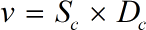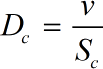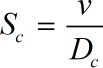Request a Tool

Schmidt Number Calculator

The Schmidt Number is used to characterize fluid flows in which there are simultaneous momentum and mass diffusion convection processes.

Output

Kinematic Viscosity
0

Formula• v = Kinematic Viscosity
• Dc = Mass Diffusivity
• Sc = Schmidt Number

Defination / Uses

The Schmidt Number is named after Ernst Heinrich Wilhelm Schmidt, a German engineer (1892-1975). The ratio of momentum diffusivity or viscosity to mass diffusivity is known as the Schmidt number, which is a dimensionless quantity. The symbol "Sc" stands for the Schmidt Number. The Schmidt Number is proportional to the thickness of the hydrodynamic and mass-transfer boundary layers.

Mass Diffusivity
0

Formula• Dc = Mass Diffusivity
• v = Kinematic Viscosity
• Sc = Schmidt Number

Defination / Uses

The Schmidt Number is named after Ernst Heinrich Wilhelm Schmidt, a German engineer (1892-1975). The ratio of momentum diffusivity or viscosity to mass diffusivity is known as the Schmidt number, which is a dimensionless quantity. The symbol "Sc" stands for the Schmidt Number. The Schmidt Number is proportional to the thickness of the hydrodynamic and mass-transfer boundary layers.

Schmidt Number
0

Formula• Sc = Schmidt Number
• Dc = Mass Diffusivity
• v = Kinematic Viscosity

Defination / Uses

The Schmidt Number is named after Ernst Heinrich Wilhelm Schmidt, a German engineer (1892-1975). The ratio of momentum diffusivity or viscosity to mass diffusivity is known as the Schmidt number, which is a dimensionless quantity. The symbol "Sc" stands for the Schmidt Number. The Schmidt Number is proportional to the thickness of the hydrodynamic and mass-transfer boundary layers.

How to use schmidt number calcultor?

There are only two basic steps.

• First select the option from dropdown. What you need to calculate.
• Then add the other values in their corresponding fields.